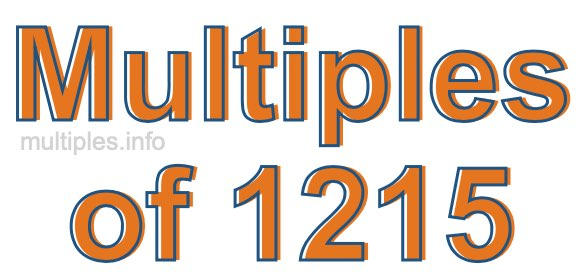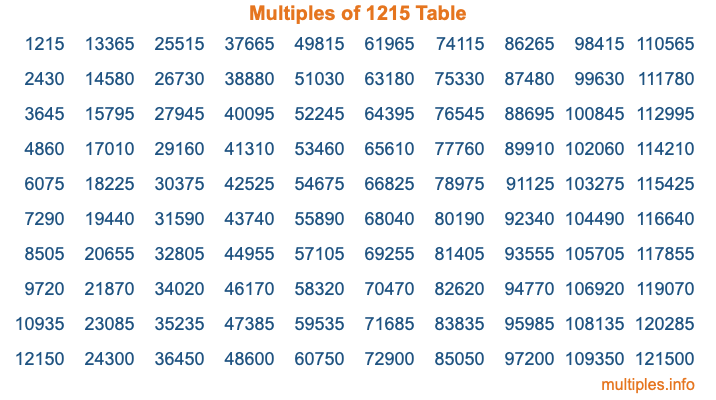Multiples of 1215Welcome to the Multiples of 1215 page. Here we will first teach you everything you will ever need to know about the multiples of 1215, and then give you a study guide summary of everything we taught you to make sure you remember it all. Use this page to look up facts and learn information about the multiples of 1215. This page will make you a multiples of one thousand two hundred fifteen expert!

Definition of Multiples of 1215
Multiples of 1215 are all the numbers that when divided by 1215 equal an integer. Each of the multiples of 1215 are called a multiple. A multiple of 1215 is created by multiplying 1215 by an integer.

Therefore, to create a list of multiples of 1215, you start with 1 multiplied by 1215, then 2 multiplied by 1215, then 3 multiplied by 1215, and so on for as long as you want. Thus, the list of the first five multiples of 1215 is 1215, 2430, 3645, 4860, and 6075. To see a larger list of multiples of 1215, see the printable image of Multiples of 1215 further down on this page. We also have a category where you can choose any nth multiple of 1215.

Multiples of 1215 Checker
The Multiples of 1215 Checker below checks to see if any number of your choice is a multiple of 1215. In other words, it checks to see if there is any number (integer) that when multiplied by 1215 will equal your number. To do that, we divide your number by 1215. If the the quotient is an integer, then your number is a multiple of 1215.

Is  a multiple of 1215?

Least Common Multiple of 1215 and ...
A Least Common Multiple (LCM) is the lowest multiple that two or more numbers have in common. This is also called the smallest common multiple or lowest common multiple and is useful to know when you are adding our subtracting fractions. Enter one or more numbers below (1215 is already entered) to find the LCM.

Check out our LCM Calculator if you need more details about the Least Common Multiple or if you need the LCM for different numbers for adding and subtraction fractions.

nth Multiple of 1215
As we stated above, 1215 is the first multiple of 1215, 2430 is the second multiple of 1215, 3645 is the third multiple of 1215, and so on. Enter a number below to find the nth multiple of 1215.

th multiple of 1215

Multiples of 1215 vs Factors of 1215
1215 is a multiple of 1215 and a factor of 1215, but that is where the similarities end. All postive multiples of 1215 are 1215 or greater than 1215. All positive factors of 1215 are 1215 or less than 1215.

Below is the beginning list of multiples of 1215 and the factors of 1215 so you can compare:

Multiples of 1215: 1215, 2430, 3645, 4860, 6075, etc.

Factors of 1215: 1, 3, 5, 9, 15, 27, 45, 81, 135, 243, 405, 1215

As you can see, the multiples of 1215 are all the numbers that you can divide by 1215 to get a whole number. The factors of 1215, on the other hand, are all the whole numbers that you can multiply by another whole number to get 1215.

It's also interesting to note that if a number (x) is a factor of 1215, then 1215 will also be a multiple of that number (x).

Multiples of 1215 vs Divisors of 1215
The divisors of 1215 are all the integers that 1215 can be divided by evenly. Below is a list of the divisors of 1215.

Divisors of 1215: 1, 3, 5, 9, 15, 27, 45, 81, 135, 243, 405, 1215

The interesting thing to note here is that if you take any multiple of 1215 and divide it by a divisor of 1215, you will see that the quotient is an integer.

Multiples of 1215 Table
Below is an image of the first 100 multiples of 1215 in a table. The table is in chronological order, column by column. The first column has the first ten multiples of 1215, the second column has the next ten multiples of 1215, and so on.The Multiples of 1215 Table is also referred to as the 1215 Times Table or Times Table of 1215. You are welcome to print out our table for your studies.

Negative Multiples of 1215
Although not often discussed or needed in math, it is worth mentioning that you can make a list of negative multiples of 1215 by multiplying 1215 by -1, then by -2, then by -3, and so on, to get the following list of negative multiples of 1215:

-1215, -2430, -3645, -4860, -6075, etc.

Multiples of 1215 Summary
Below is a summary of important Multiples of 1215 facts that we have discussed on this page. To retain the knowledge on this page, we recommend that you read through the summary and explain to yourself or a study partner why they hold true.

There are an infinite number of multiples of 1215.

A multiple of 1215 divided by 1215 will equal a whole number.

1215 divided by a factor of 1215 equals a divisor of 1215.

The nth multiple of 1215 is n times 1215.

The largest factor of 1215 is equal to the first positive multiple of 1215.

1215 is a multiple of every factor of 1215.

1215 is a multiple of 1215.

A multiple of 1215 divided by a divisor of 1215 equals an integer.

1215 divided by a divisor of 1215 equals a factor of 1215.

Any integer times 1215 will equal a multiple of 1215.

Multiples of a Number
Here you can get the multiples of another number, all with the same attention to detail as we did for multiples of 1215 on this page.

Multiples of
Multiples of 1216
Did you find our page about multiples of one thousand two hundred fifteen educational? Do you want more knowledge? Check out the multiples of the next number on our list!